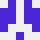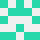# 如何编写 Lua 模式将字符串（嵌套数组）转换为真正的数组？

``````function stringToArray()
local Array = {}
local txt = "{ {Group = 123, Pos = 200}, {Group = 124, Pos = 205} }"
for str in string.gmatch(txt, .....
end
``````stackoverflow用户1514861

``````local txt = "{ {Group = 123, Pos = 200}, {Group = 124, Pos = 205} }"
local Array = load("return " .. txt)()
print(Array.Group) --输出 123
``````

2022-03-03 10:51:22stackoverflow用户11740758

``````local txt = "{ {Group = 123, Pos = 200}, {Group = 124, Pos = 205} }"
local _, lcurlies = txt:gsub('%{','')
local _, rcurlies = txt:gsub('%}','')
local _, commas = txt:gsub(',','')
local _, spaces = txt:gsub('%s','')
local _, equals = txt:gsub('=','')

print(lcurlies,rcurlies,commas,spaces,equals)
-- 输出：3 3 3 13 4
``````
2022-03-05 22:37:24